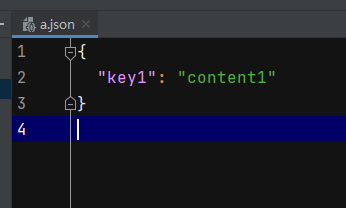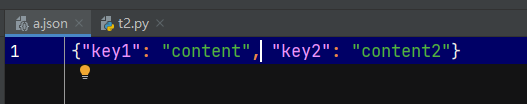• Python txt文件读取写入字典的方法 一、json方式 1、字典写入txt import json dic = { 'andy':{ 'age': 23, 'city': 'beijing', 'skill': 'python' }, 'william': { 'age': 25, 'city': 'shanghai'...
Python txt文件读取写入字典的方法
一、json方式
1、字典写入txt
import json

dic = {
'andy':{
'age': 23,
'city': 'beijing',
'skill': 'python'
},
'william': {
'age': 25,
'city': 'shanghai',
'skill': 'js'
}
}

js = json.dumps(dic)
file = open('test.txt', 'w')
file.write(js)
file.close()

2、读取txt中的字典
import json

file = open('test.txt', 'r')
js = file.read()
dic = json.loads(js)
print(dic)
file.close()

二、使用str转换方法
1、字典写入txt
dic = {
'andy':{
'age': 23,
'city': 'beijing',
'skill': 'python'
},
'william': {
'age': 25,
'city': 'shanghai',
'skill': 'js'
}
}

fw = open("test.txt",'w+')
fw.write(str(dic))      #把字典转化为str
fw.close()

2、读取txt中字典
fr = open("test.txt",'r+')
dic = eval(fr.read())   #读取的str转换为字典
print(dic)
fr.close()

一个转换程序：
-- coding: UTF-8 --
import json
import collections
import io
filename = "/Users/liuhaojie01/Desktop/landmark.txt"
print(filename)
#detail = collections.defaultdict(int)
#agelist = []
#with io.open(filename, 'r', encoding = 'utf-8') as file_to_read:
##    lines = file_to_read.readline()  # 整行读取数据
#    str = file_to_read.read()

file = open(filename, 'r')
js = file.read()
dic = json.loads(js)

print(len(dic['data']))
print(len(dic['data']))
with open("/Users/liuhaojie01/Desktop/landmarkdata.txt","w+") as f:
for frame in dic["data"]: #每一帧
data  =[]
for dicdata in frame:
print(dicdata["x"],dicdata["y"])
data.append(str(dicdata["x"]))
data.append(" ")
data.append(str(dicdata["y"]))
data.append(" ")

#    print(len(i),type(i))
f.writelines(data)
f.write("\n")
file.close()
f.close()



展开全文json
• Python txt文件读取写入字典的方法（json、eval） 一、使用json转换方法 1、字典写入txt import json dic = { 'andy':{ 'age': 23, 'city': 'beijing', 'skill': 'python' }, 'william': { 'age': 25, '...
                         Python txt文件读取写入字典的方法（json、eval）

一、使用json转换方法
1、字典写入txt

import json

dic = {
'andy':{
'age': 23,
'city': 'beijing',
'skill': 'python'
},
'william': {
'age': 25,
'city': 'shanghai',
'skill': 'js'
}
}

js = json.dumps(dic)
file = open('test.txt', 'w')
file.write(js)
file.close() 

2、读取txt中的字典

import json

file = open('test.txt', 'r')
js = file.read()
dic = json.loads(js)
print(dic)
file.close()

二、使用str转换方法
1、字典写入txt

dic = {
'andy':{
'age': 23,
'city': 'beijing',
'skill': 'python'
},
'william': {
'age': 25,
'city': 'shanghai',
'skill': 'js'
}
}

fw = open("test.txt",'w+')
fw.write(str(dic)) #把字典转化为str
fw.close()

2、读取txt中字典

fr = open("test.txt",'r+')
dic = eval(fr.read()) #读取的str转换为字典
print(dic)
fr.close()


展开全文• f = open('pyehon测试....#不加参数时，open为你默认为'r'，reading，只读模式，不能写入文件必须存在，否则引发异常。 data = f.read();#读取文件中的所有内容 data2=f.readline();#读取一行内容 data3=f.read...


f = open('pyehon测试.txt');#py2里可以使用 open 或 file 方法打开文件，py3 只能使用 open。
#不加参数时，open为你默认为'r'，reading，只读模式，不能写入且文件必须存在，否则引发异常。
data = f.read();#读取文件中的所有内容
data2=f.readline();#读取一行内容
data3=f.readlines();#把内容按行读取至一个list中
f.close();
d = open('pyehon测试2.txt','w');#'w'就是writing，以这种模式打开文件，原来文件中的内容会被你新写入的内容覆盖掉，如果文件不存在，会自动创建文件。
h = open('pyehon测试3.txt','a');#'a'就是appending。它也是一种写入模式，但你写入的内容不会覆盖之前的内容，而是添加到文件中
#'w'和'a'模式不可以读取，只能写入
print(data);
h.write(data);#写入内容
h.close();
d.close();

#break 跳出循环
#continue 略过本次循环
#异常处理 try ... except
try:
i = 'sd';
print(i+10);
except:
print("出现错误");

#字典
d ={};#创建空字典
d["MyLove"] = "YanHe";#增加新键
d = 'kdjf';#如果你的键是字符串，通过键访问的时候就需要加引号，如果是数字作为键则不用。
del d;#删除键值
d["MyLove"] = "LuoTianYi";#更改键值

#引用模块
import random;   #调用模块，类似于using+类名
random.randint(1,10);#使用模块中的函数
dir(random); #查询random里面有哪些函数

from math import pi as math_pi; #从math模块里面调用pi函数命名为math_pi
print(math_pi);

转载于:https://www.cnblogs.com/LoveYanHe/p/8877919.html
展开全文• YDOOK：JSON：Python 读写读取写入 同时读写 json文件完全教程 1. 使用：str = json.dumps (Python_obj) ： 将 Python_obj Python对象 转变为=> : str 字符串 2. 使用：json_obj = json.dumps (Python_str) ： 将...
YDOOK：JSON：Python 读写读取写入 同时读写  json文件完全教程
1. 使用：str = json.dumps (Python_obj) ：
将 Python_obj Python对象 转变为=> : str 字符串
2. 使用：json_obj = json.dumps (Python_str) ：
将 Python_str 字符串 转变为=> : json_obj json对象字典

3. 将字符串转变为 Python对象时，转变的对象的表现形式为：Python字典。

4. 现在，新建一个 a.json文件：{
"key1": "content1"
}



4. Python 读取 a.json文件内容：
import json

with open("./a.json", "r+") as f:

python_dict = json.load(f)
print('python_dict = ', python_dict)
print('type(python_dict) = ', type(python_dict))


注意：读写格式必须是 “r” 或者 “r+”, 否则会导致被读取的 json文件清空。

5. Python 同时读写 读取写入 a.json文件内容：
import json

# Read ©YDOOK JYLin
with open("./a.json", "r+") as f:

python_dict = json.load(f)
print('python_dict = ', python_dict)
print('type(python_dict) = ', type(python_dict))
python_dict["key2"] = "content2"
print('python_dict = ', python_dict)
print('type(python_dict) = ', type(python_dict))
f.seek(0)  # 这两行代买顺序不能变化，用于清空原文件内容
f.truncate() # 这两行代买顺序不能变化，用于清空原文件内容
json.dump(python_dict, f)
f.close()


6. 操作后，a.json 文件内容变为：
{"key1": "content", "key2": "content2"}展开全文读写JSON
• 需求： 因需要将一json文件中大量的信息填入一固定格式的Excel...解决思路是，读取json文件，然后遍历字典的键和值，读完第一个字典写入Excel后换行，读取第二个字典。 代码： # -*- coding: utf-8 -*- import xlwt
• #持久化保存： 文件 list 元组 字典---->内存 #用户注册 def register(): username=input('输入用户名：') password=input('输入密码：') repassword=input('再次确认密码：') if password==repassword : #...
• 1、先写一下Python中的路径 分隔符 ①用斜杠（/）表示， ②也可以用反斜杠（\）标识：由于反斜杠也用作转义字符，所以要用双反斜杠（\\）来分隔路径；也可以使用r‘’+单反斜杠 表示路径，r指明是原始字符串，...os模块
• 一、yaml文件介绍 yaml是一个专门用来写配置文件的语言。 1. yaml文件规则 区分大小写； 使用缩进表示层级关系；... 使用空格键缩进，而非Tab键缩进 ...对象：键值对的集合（简称 "映射或字典"） 键值...
• 1、由于python默认读取write()写入read()文件的数据格式为字符串（str），不能读取写入列表、字典等类型数据，所以需要采用json数据格式来存储数据。 2、json数据格式在多种语言中通用，有利于分享数据。 二、python...json
• Python 把嵌套字典写入文件，并读取出来 直接上代码 写入 del str file = open('example.txt', 'w') for k,v in dict.items(): file.write(k + ' ') for k1, v1 in v.items(): file.write(k1 + ' ' + str(v1) + '...
• json dumps把数据类型转换成字符串 dump把数据类型转换成字符串并存储在文件中 loads把字符串转换成数据类型 load把文件打开从字符串转换成数据类型 json是可以在不同语言之间交换数据的，而pickle只在python之间...
• 方法一: 使用pandas 写入csv文件 import pandas as pd #任意的多组列表 a = [&quot;a&quot;, &quot;b&quot;, &quot;c&quot;] b = [&quot;d&quot;, &quot;e&quot;, &...csv pandas mongo...

# python文件读取写入字典python 订阅